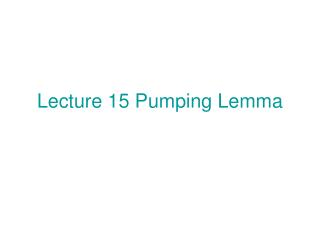DownloadDownload PresentationLecture 15 Pumping Lemma

# Lecture 15 Pumping Lemma

Télécharger la présentation## Lecture 15 Pumping Lemma

- - - - - - - - - - - - - - - - - - - - - - - - - - - E N D - - - - - - - - - - - - - - - - - - - - - - - - - - -
##### Presentation Transcript

1. Lecture 15 Pumping Lemma

2. Consider a CFG G = (V, Σ, R, S). Assume for any rule A → w in R, |w| < c for a constant c > 0. For x in L(G), consider a parse tree T for x. s > logc |x| x This tree would have at least |x| leaves. Therefore, the depth of this tree > logc |x|

3. |v|+1 Let |x| > K = c . Then the depth of parse tree T > |V|+1. So, there is a path from root to a leaf with length > |V|+1, That is, it contains at least |V|+1 internal nodes. s x Thus, there exists a nonterminal symbol, say A appearing twice.

4. Tree T is decomposed into three smaller trees I, II, III. S S S I A I u z A A A z u u z III v y II A A w y v y v III w w Corresponding to this tree decomposition, x = uvwyz which has property that for any i > 0 uv wy z L(G). i i

5. To make this property significant, we should have vy ≠ ε . Choose T to be a parse tree for x with minimum number of nodes. Then we would have vy ≠ ε. If vy = ε, then S I A u v III w is a parse tree for x with less number of node than T. (-> <-)

6. Pumping Lemma (weak): For any CFL L, there exists a Constant K > 0 such that any x L with |x| > K can be Decomposed as x = uvwyz satisfying (1) vy ≠ ε, (2) for any i > 0, uv wy z L. i i It is similar to the situation od pumping lemma fo regular languages that this lemma may not easy to use. Therefore, we would like to add some condition which can restrict the location of v and y. The following is such A condition. (3) |vwy| < K.

7. To get condition (3), choose A to be the lowest one among nonterminal symbols appearing again in the subpath from which to a leaf. S I A u z II A < |V|+1 y v III w Therefore subtree II+III has depth < |V|+1. Hence, it Has at most K=c leaves, i.e., (3) holds. |V|+1

8. Pumping Lemma (strong): For any CFL L, there exists a Constant K > 0 such that any x L with |x| > K can be Decomposed as x = uvwyz satisfying (1) vy ≠ ε, (2) for any i > 0, uv wy z L, (3) |vwy| < K. i i

9. n n n Example 1 L={a b c | n > 0} is not CF.

10. CFL is not closed under intersection! m m n A = {a b c | m, n > 0} B = {a b c | m, n > 0} m n n Both A and B are CFL, but A ∩ B = {a b c | n > 0} is not. n n n

11. Example 2. L={ww | w (0+1)*} is not CF.

12. CFL is not closed under complement! For L = {ww | w (0+1)*}, L is a CFL. However, L = L is not.

13. L={0 | n is a prime} is not regular. n Proof. For contradiction, suppose L is CF. Let K > 0 be the constant in Pumping Lemma. Consider a prime p > K. Then, 0 = uvwxy such that vx≠ε and for any i > 0 uv wx y is in L. Thus, |u| + |w| + |y| + i(|v|+|x|) is a prime for any I > 0. p i i

14. For i =0, |u|+|w| + |y| is a prime. For i =|u|+|w|+|y|, |u|+|w|+|y|+ i(|v|+|x|) = (|u|+|w|+|y|)(1+|v|+|x|) is a prime. (-><-)

15. Theorem For a language L with one symbol, L is CF if and only if L is regular.